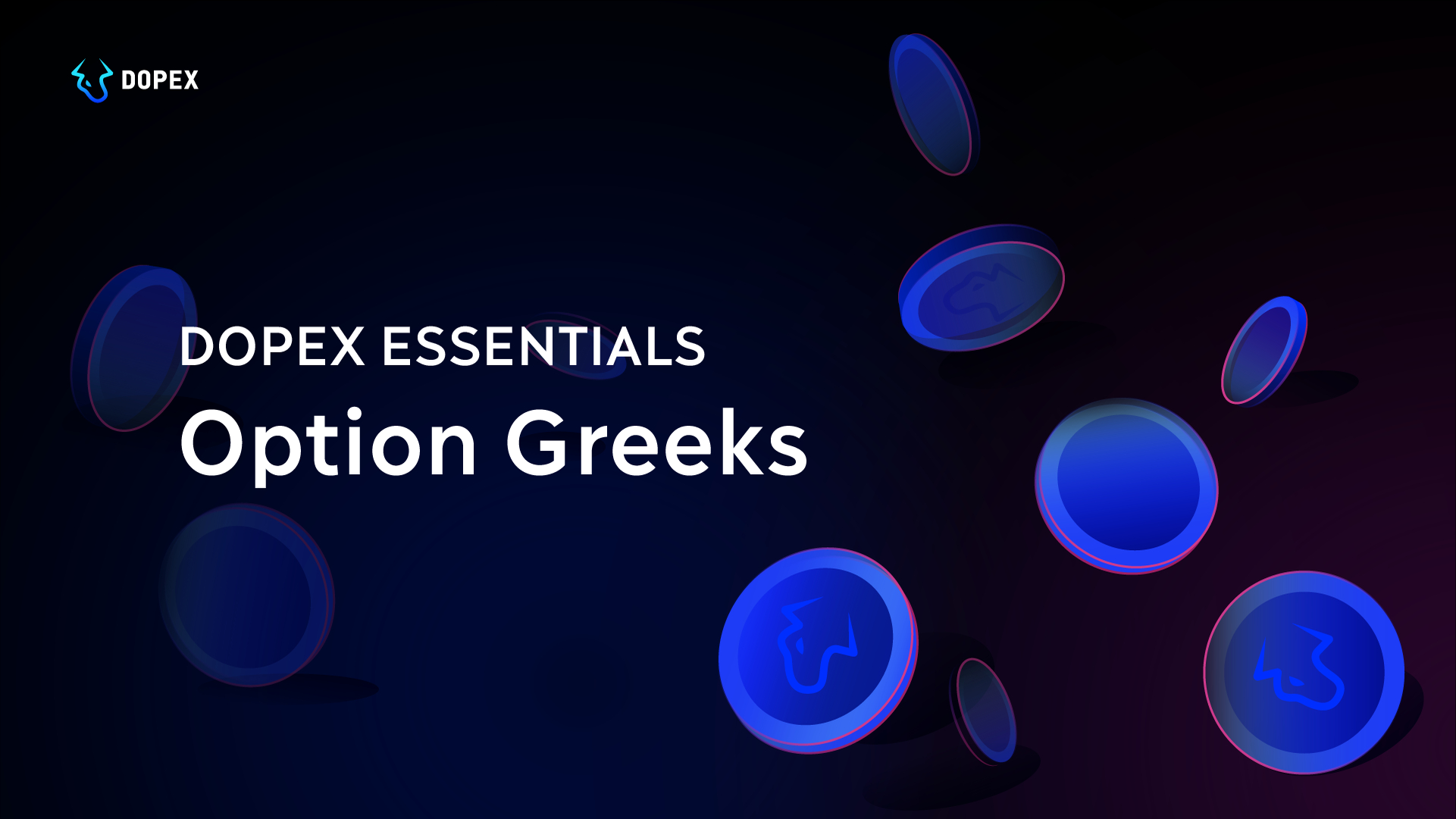# Dopex Essentials: Option Greeks (A Brief Overview)

Dopex-Essentials# Key Points:

• Delta is inclined to increase closer to expiration for near or at-the-money options.
• Delta is further evaluated by gamma, which is a measure of delta’s rate of change.
• Delta can also change in reaction to implied volatility changes.

# Key Points

• The value of gamma is lower for deep out-of-the-money and deep-in-the-money options.
• The value of gamma is highest when the option gets near the money.
• The value of gamma is positive for long options and negative for short options.

# Key Points:

• OTM options with a lot of implied volatility tend to have high values of Theta.
• Theta is typically highest for at-the-money options since less time is needed to earn a profit with a price move in the underlying asset.
• Theta will increase sharply as time decay accelerates in the last few weeks before expiration and can severely undermine a long option holder’s position, especially if implied volatility decreases at the same time.

# Key Points:

• Due to changes in implied volatility, the value of vega can fluctuate even without price changes to the underlying asset.
• Vega can increase in reaction to sudden changes in the price of the underlying asset.
• As the option gets closer to the expiration date, the value of vega decreases.

# Glossary of terms

###### In the money (ITM):
• For a call — this term is used when the strike price is lower than the current price of the underlying asset.
• For a put — this term is used when the strike is higher than the current price.
###### At the money (ATM):
• For both a call and a put — this term is used when the strike is equal to the current price.
###### Out of the money (OTM):
• For a call — this term is used when the strike price is higher than the current price of the underlying asset.
• For a put — this term is used when the strike is lower than the current price.

← Back to Blog